# Constrained Nonlinear Curve Fit VI

## LabVIEW 2018 Help

Edition Date: March 2018
Part Number: 371361R-01
View Product Info

### DOWNLOAD (Windows Only)LabVIEW 2016 HelpLabVIEW 2017 HelpLabVIEW 2018 HelpLabVIEW 2019 HelpLabVIEW 2020 Help

Owning Palette: Fitting VIs

Requires: Full Development System

Uses either the Levenberg-Marquardt algorithm or the trust-region dogleg algorithm to determine the set of parameters that best fit the set of input data points (X, Y) as expressed by a nonlinear function y = f(x,a), where a is the set of parameters. You must manually select the polymorphic instance to use.

Use the pull-down menu to select an instance of this VI.

 Select an instance Nonlinear Curve Fit LM boundNonlinear Curve Fit TRDL bound

## Nonlinear Curve Fit LM bound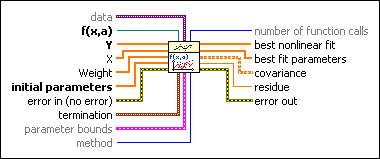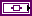data specifies static data that the user-defined function needs at run time.f(x,a) is a reference to the VI that implements the fitting model. a is the set of parameters LabVIEW calculates. Use the VI template located at labview\vi.lib\gmath\NumericalOptimization\LM model function and gradient.vit to create the VI from a template.Y specifies the array of dependent values. The number of input points must be greater than zero and greater than the number of initial parameters. The number of elements in Y must be equal to the number of elements in X.X specifies the array of independent values. The number of input points must be greater than zero and greater than the number of initial parameters. The number of elements in X must be equal to the number of elements in Y.Weight is the array of weights for the observations Y. If Weight is unwired, this VI sets all elements of Weight to 1. If Weight has fewer elements than Y, this VI pads the end of Weight with ones so that the length of Weight equals the length of Y. If Weight has more elements than Y, this VI ignores the extra elements at the end of Weight. If an element in Weight is less than 0, this VI uses the absolute value of the element.initial parameters specifies the initial guess for a solution. The success of the nonlinear curve fit depends on how close the initial parameters are to the solution. Therefore, use any available resources to obtain good initial guess coefficients to the solution before you use this VI.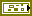error in describes error conditions that occur before this node runs. This input provides standard error in functionality.termination specifies the stopping conditions for the fitting process.max iteration specifies the largest number of iterations of the fitting routine. If the number of iterations exceeds max iterations, the fitting process terminates.tolerance specifies the relative change in the weighted distance between Y and the current fit. If the relative change falls below tolerance, the fitting process terminates.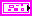parameter bounds is a cluster that contains the upper and lower numeric limits for the parameters being optimized.parameter maximum contains the highest allowed value of the parameters being optimized. The array does not accept values of NaN. The size of this array must either equal the size of the initial parameters array or be 0. For all i, parameter maximum[i] must be greater than or equal to parameter minimum[i]. If parameter maximum[i] equals parameter minimum[i], LabVIEW uses that constant value throughout the curve fitting.parameter minimum contains the lowest allowed value of the parameters being optimized. The array does not accept values of NaN. The size of this array must either equal the size of the initial parameters array or be 0.method specifies the fitting method.

 0 Least Square (default) 1 Least Absolute Residual 2 Bisquare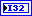number of function calls returns the number of times LabVIEW called f(x,a) during the fitting process.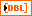best nonlinear fit returns the y-values of the fitted model that correspond to the independent values in X.best fit parameters returns the array of parameters that minimizes the weighted mean square error between the solution vector and the observed y-values.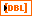covariance returns the matrix of covariances. Cjk is the covariance between a[j] and a[k]. c[jj] is the variance of a[j]. This VI generates the covariance, C, according to the following equation:

C = (1/2)D^–1

where D is the Hessian of the function with respect to its parameters.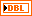residue returns the weighted mean square error between the best nonlinear fit and Y.error out contains error information. This output provides standard error out functionality.

## Nonlinear Curve Fit TRDL boundNote  Uses the trust-region dogleg algorithm to determine the set of parameters that best fit the set of input data points (X, Y) as expressed by a nonlinear function y = f(x,a), where a is the set of parameters.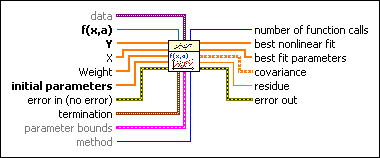data specifies static data that the user-defined function needs at run time.f(x,a) is a reference to the VI that implements the fitting model. a is the set of parameters LabVIEW calculates. Use the VI template located at labview\vi.lib\gmath\NumericalOptimization\LM model function and gradient.vit to create the VI from a template.Y specifies the array of dependent values. The number of input points must be greater than zero and greater than the number of initial parameters. The number of elements in Y must be equal to the number of elements in X.X specifies the array of independent values. The number of input points must be greater than zero and greater than the number of initial parameters. The number of elements in X must be equal to the number of elements in Y.Weight is the array of weights for the observations Y. If Weight is unwired, this VI sets all elements of Weight to 1. If Weight has fewer elements than Y, this VI pads the end of Weight with ones so that the length of Weight equals the length of Y. If Weight has more elements than Y, this VI ignores the extra elements at the end of Weight. If an element in Weight is less than 0, this VI uses the absolute value of the element.initial parameters specifies the initial guess for a solution. The success of the nonlinear curve fit depends on how close the initial parameters are to the solution. Therefore, use any available resources to obtain good initial guess coefficients to the solution before you use this VI.error in describes error conditions that occur before this node runs. This input provides standard error in functionality.termination specifies the stopping conditions for the fitting process.max iteration specifies the largest number of iterations of the fitting routine. If the number of iterations exceeds max iterations, the fitting process terminates.tolerance specifies the relative change in the weighted distance between Y and the current fit. If the relative change falls below tolerance, the fitting process terminates.parameter bounds is a cluster that contains the upper and lower numeric limits for the parameters being optimized.parameter maximum contains the highest allowed value of the parameters being optimized. The array does not accept values of NaN. The size of this array must either equal the size of the initial parameters array or be 0. For all i, parameter maximum[i] must be greater than or equal to parameter minimum[i]. If parameter maximum[i] equals parameter minimum[i], LabVIEW uses that constant value throughout the curve fitting.parameter minimum contains the lowest allowed value of the parameters being optimized. The array does not accept values of NaN. The size of this array must either equal the size of the initial parameters array or be 0.method specifies the fitting method.

 0 Least Square (default) 1 Least Absolute Residual 2 Bisquarenumber of function calls returns the number of times LabVIEW called f(x,a) during the fitting process.best nonlinear fit returns the y-values of the fitted model that correspond to the independent values in X.best fit parameters returns the array of parameters that minimizes the weighted mean square error between the solution vector and the observed y-values.covariance returns the matrix of covariances. Cjk is the covariance between a[j] and a[k]. c[jj] is the variance of a[j]. This VI generates the covariance, C, according to the following equation:

C = (1/2)D^–1

where D is the Hessian of the function with respect to its parameters.residue returns the weighted mean square error between the best nonlinear fit and Y.error out contains error information. This output provides standard error out functionality.

Not Helpful